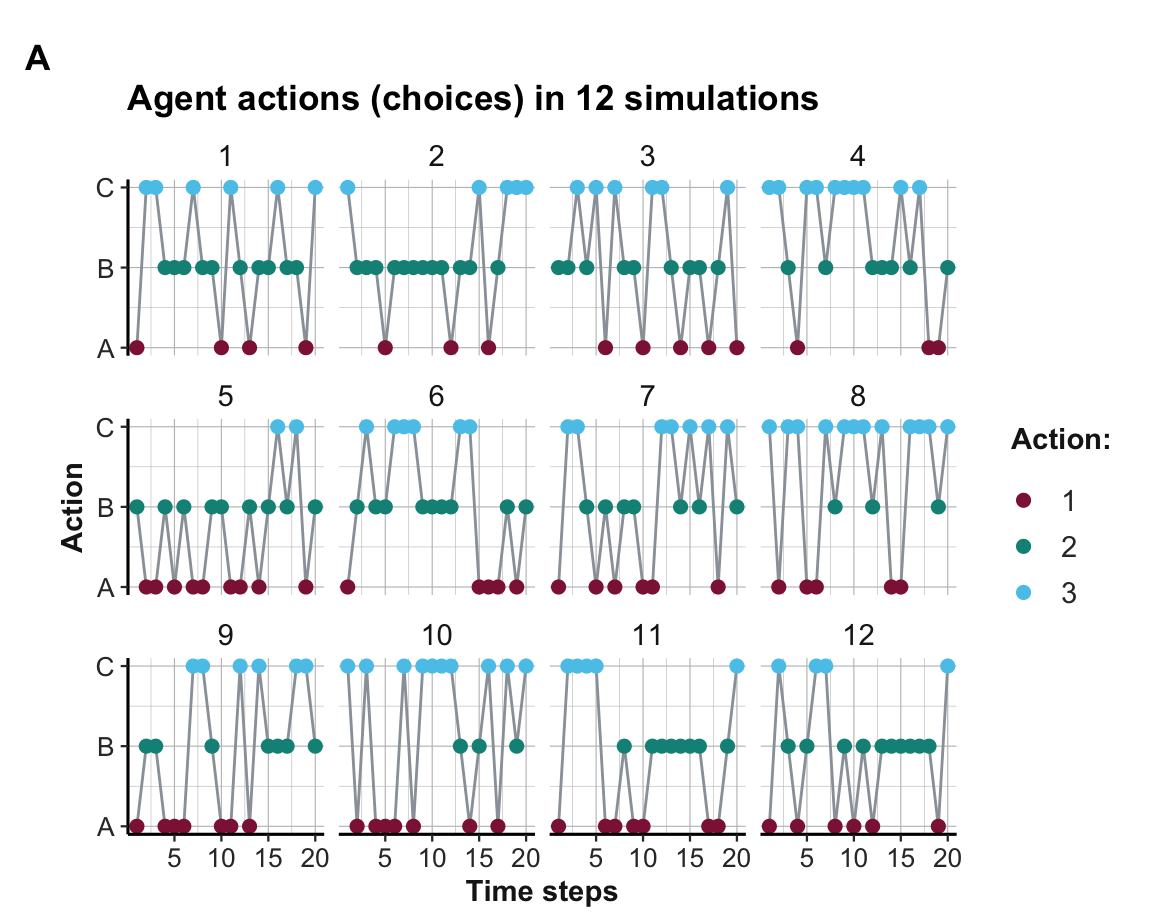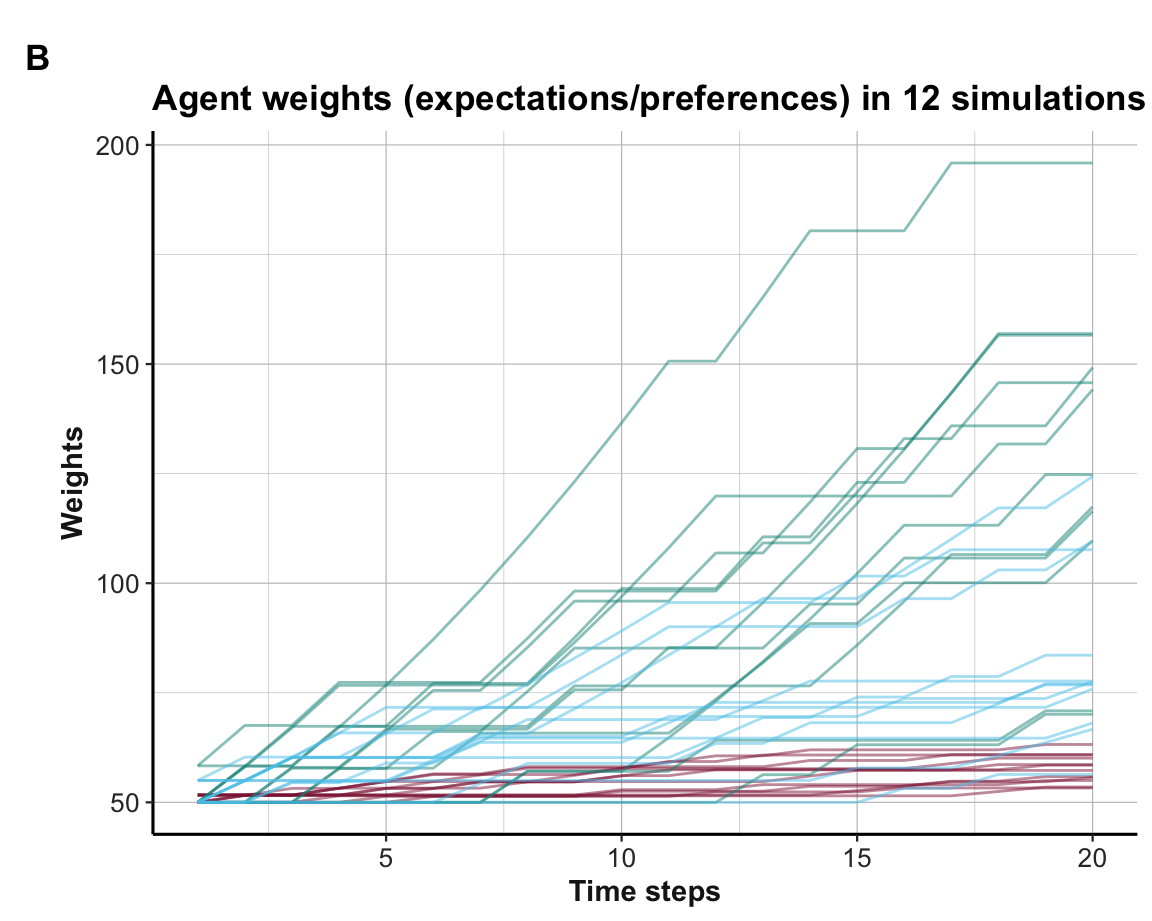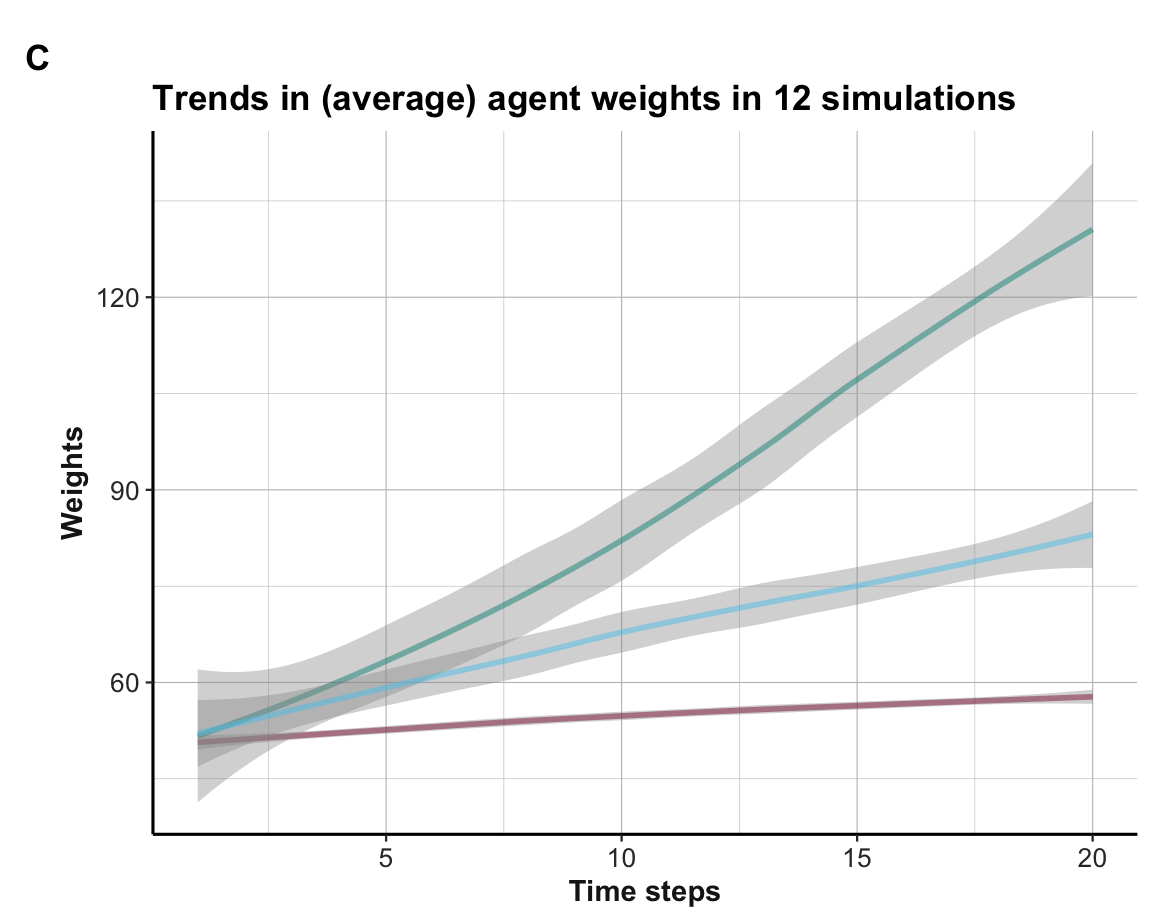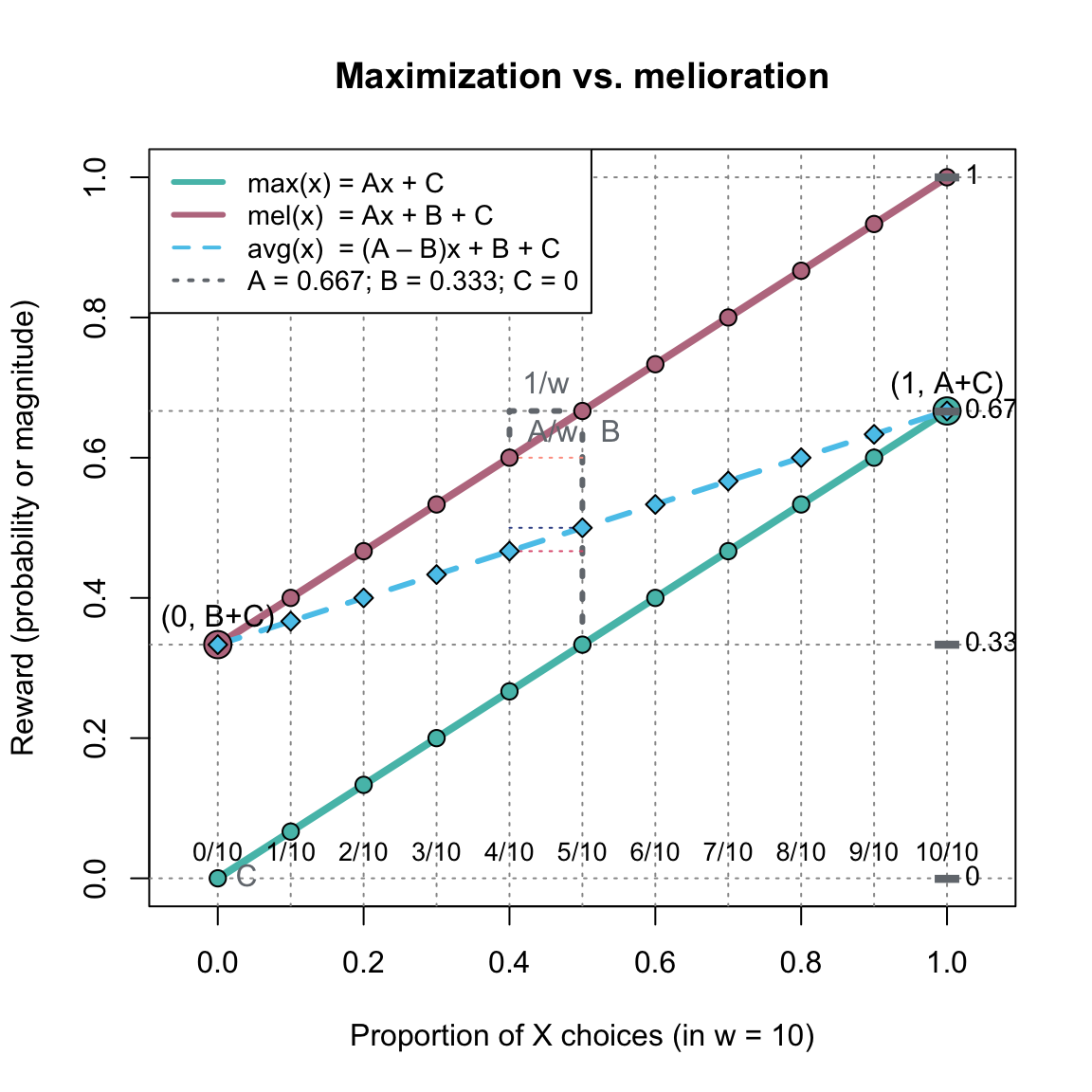## 17.6 ExercisesHere are some exercises for simple learning agents that interact with stable or dynamic environments.

### 17.6.1 Learning with short-term aspirations

Our basic learning model (developed in Section 17.2) assumed that a learning agent has a constant aspiration level of $$A = 5$$. As the reward from selecting the worst option still exceeds $$A$$, this always leads to a positive “surprise” upon receiving a reward, corresponding increments of $$\Delta w > 0$$, and monotonic increases in weight values wgt. Although having low expectations may guarantee a happy agent, it seems implausible that an agent never adjusts its aspiration level $$A$$. This exercise tries the opposite approach, by assuming an extremely myopic agent who always updates $$A$$ to the most recent reward value:

1. Adjust $$A$$ to the current reward value on each trial $$t$$.

Hint: This also requires that the current reward value is explicitly represented in each loop, rather than only being computed implicitly when calling the delta_w() function.

1. Does the agent still successfully learn to choose the better option? Describe how the learning process changes (in terms of the values of $$A$$ and $$wgt$$, and the options being chosen).

2. Given the new updating mechanism for $$A$$ and assuming no changes in the agent’s learning rate alpha, the initial wgt values and delta_w(), how could a change in the probability of choosing options (i.e., the p() function) increase the speed of learning?

3. Explain why it is essential that $$A$$ is adjusted after updating the agent’s wgt values. What would happen, if an agent prematurely updated its aspiration level to the current reward (prior to updating wgt)?

#### Solution

• ad 1.: We copy the functions p(), r() and delta_w(), as defined in Section 17.2:
# 1. Choosing:
p <- function(k){  # Probability of k:

wgt[k]/sum(wgt)  # wgt denotes current weights

}

# Reward from choosing k:
r <- function(k){

rew[k]  # rew denotes current rewards

}

# 2. Learning:
delta_w <- function(k){  # Adjusting the weight of k:

(alpha * p(k) * (r(k) - A))

}

the following code extends the code from above in two ways:

• After updating wgt, the current reward value cur_rew is explicitly determined (for a second time) and the aspiration level A is set to this value on each individual trial (see Step 3 within the inner loop);

• data is extended to include the current value of A (or cur_rew) on each individual trial.

# Simulation:
n_S <- 12  # number of simulations
n_T <- 20  # time steps/cycles (per simulation)

# Environmental constants:
alt <- c("A", "B")  # constant options
rew <- c(10, 20)    # constant rewards (A < B)

# Prepare data structure for storing results:
data <- as.data.frame(matrix(ncol = (4 + length(alt)), nrow = n_S * n_T))
names(data) <- c("s", "t", "act", "A", paste0("w_", alt))

for (s in 1:n_S){     # each simulation: ----

# Initialize agent:
alpha <- 1        # learning rate
A <- 5            # aspiration level
wgt <- c(50, 50)  # initial weights

for (t in 1:n_T){   # each step/trial: ----

# (1) Use wgt to determine current action:
cur_prob <- c(p(1), p(2))
cur_act <- sample(alt, size = 1, prob = cur_prob)
ix_act <- which(cur_act == alt)

# (2) Update wgt (based on reward):
new_w <- wgt[ix_act] + delta_w(ix_act)  # increment weight
wgt[ix_act] <- new_w  # update wgt

# (3) Determine reward and adjust A:
cur_rew <- rew[ix_act]  # value of current reward
A <- cur_rew            # update A

# (+) Record results:
data[((s-1) * n_T) + t, ] <- c(s, t, A, ix_act, wgt)

} # for t:n_T end.

print(paste0("s = ", s, ": Ran ", n_T, " steps, wgt = ",
paste(round(wgt, 0), collapse = ":"),
", A = ", A))

} # for i:n_S end.
#>  "s = 1: Ran 20 steps, wgt = 34:76, A = 10"
#>  "s = 2: Ran 20 steps, wgt = 33:79, A = 10"
#>  "s = 3: Ran 20 steps, wgt = 43:66, A = 20"
#>  "s = 4: Ran 20 steps, wgt = 33:86, A = 20"
#>  "s = 5: Ran 20 steps, wgt = 37:76, A = 20"
#>  "s = 6: Ran 20 steps, wgt = 31:83, A = 10"
#>  "s = 7: Ran 20 steps, wgt = 39:72, A = 20"
#>  "s = 8: Ran 20 steps, wgt = 43:66, A = 20"
#>  "s = 9: Ran 20 steps, wgt = 30:94, A = 20"
#>  "s = 10: Ran 20 steps, wgt = 33:79, A = 10"
#>  "s = 11: Ran 20 steps, wgt = 30:94, A = 20"
#>  "s = 12: Ran 20 steps, wgt = 29:97, A = 20"

# Report result:
print(paste0("Finished running ", n_S, " simulations (see 'data' for results)"))
#>  "Finished running 12 simulations (see 'data' for results)"

The printed summary information after each simulation shows that all agents seem to successfully discriminate between options (by learning a higher weight for the superior Option B).

• ad 2.: From the 2nd trial onwards, the agent always aspires to its most recent reward. Thus, the agent is never surprised when the same reward is chosen again and only learns upon switching options:

• When switching from Option A to Option B, the reward received exceeds $$A$$, leading to an increase in the weight for Option B.

• When switching from Option B to Option A, the reward received is lower than $$A$$, leading to an decrease in the weight for Option A. This explains why the weights of Option A can decrease and generally are lower than their initial values in this simulation.

• ad 3.: Given this setup, the pace of learning depends on the frequency of switching options. Increasing the agent’s propensity for switching would increase its learning speed.

• ad 4.: If Steps 2 and 3 were reversed (i.e., A was updated to the current reward value prior to updating wgt), the difference r(k) - A in delta_w() would always be zero. Thus, the product $$\Delta w(k)$$ will always be zero, irrespective of $$k$$ or $$t$$. Overall, the premature agent would never learn anything. (Consider trying this to verify it.)

### 17.6.2 Learning with additional options

This exercise extends our basic learning model (from Section 17.2) to a stable environment with additional options:

1. Extend our learning model to an environment with three options (named A, B, and C) that yield stable rewards (of 10, 30, and 20 units, respectively).

2. Visualize both the agent choices (i.e., actions) and expectations (i.e., weights) and interpret what they show.

3. Assuming that the options and rewards remain unchanged, what could improve the learning success? (Make a prediction and then test it by running the simulation.)

#### Solution

• ad 1.: Still using the functions p(), r() and delta_w(), as defined in Section 17.2:
# 1. Choosing:
p <- function(k){  # Probability of k:

wgt[k]/sum(wgt)  # wgt denotes current weights

}

# Reward from choosing k:
r <- function(k){

rew[k]  # rew denotes current rewards

}

# 2. Learning:
delta_w <- function(k){  # Adjusting the weight of k:

(alpha * p(k) * (r(k) - A))

}

the following code extends the simulation from above to three options:

# Simulation:
n_S <- 12  # number of simulations
n_T <- 20  # time steps/cycles (per simulation)

# Environmental constants:
alt <- c("A", "B", "C")  # constant options
rew <- c(10, 30, 20)     # constant rewards

# Prepare data structure for storing results:
data <- as.data.frame(matrix(ncol = (3 + length(alt)), nrow = n_S * n_T))
names(data) <- c("s", "t", "act", paste0("w_", alt))

for (s in 1:n_S){  # each simulation: ----

# Initialize learner:
wgt <- c(50, 50, 50)  # initial weights
alpha <- 1  # learning rate
A <- 5      # aspiration level

for (t in 1:n_T){  # each step: ----

# (1) Use wgt to determine current action:
cur_prob <- c(p(1), p(2), p(3))
cur_act <- sample(alt, size = 1, prob = cur_prob)
ix_act <- which(cur_act == alt)

# (2) Update wgt (based on reward):
new_w <- wgt[ix_act] + delta_w(ix_act)  # increment weight
wgt[ix_act] <- new_w  # update wgt

# (+) Record results:
data[((s-1) * n_T) + t, ] <- c(s, t, ix_act, wgt)

} # for t:n_T end.

print(paste0("s = ", s, ": Ran ", n_T, " steps, wgt = ",
paste(round(wgt, 0), collapse = ":")))

} # for i:n_S end.
#>  "s = 1: Ran 20 steps, wgt = 55:157:78"
#>  "s = 2: Ran 20 steps, wgt = 53:196:67"
#>  "s = 3: Ran 20 steps, wgt = 56:146:77"
#>  "s = 4: Ran 20 steps, wgt = 53:110:108"
#>  "s = 5: Ran 20 steps, wgt = 63:149:56"
#>  "s = 6: Ran 20 steps, wgt = 56:144:78"
#>  "s = 7: Ran 20 steps, wgt = 59:116:84"
#>  "s = 8: Ran 20 steps, wgt = 57:71:124"
#>  "s = 9: Ran 20 steps, wgt = 61:117:77"
#>  "s = 10: Ran 20 steps, wgt = 61:70:110"
#>  "s = 11: Ran 20 steps, wgt = 60:125:76"
#>  "s = 12: Ran 20 steps, wgt = 58:157:68"
• ad 2.: As before, the data frame data collects information on all intermediate states. This allows us to visualize the learning process in terms of the actions chosen (choices):Figure 17.9: Options chosen (over 12 simulations).

internal expectations (weight values):Figure 17.10: Weight values (over 12 simulations).

and their average trends:Figure 17.11: Average trends in weight values.

#### Interpretation

The plot of agent actions (A) shows that some, but not all agents learn to choose the best Option B within 20 rounds. Due to the more complicated setup of three options and their more similar rewards, the learning is slower and not always successful. This is also reflected in the visualization of the weights (shown in B), which are not separated as cleanly as in the binary case (see Section 17.2). However, the average trends (shown in C) still provide systematic evidence for learning when averaging the agent weight values over all 12 simulations.

• ad 3.: Increasing the learning rate (e.g., to $$\alpha = 2$$) or increasing the number of trials $$n_T$$ per simulation both lead to better (i.e., faster or more discriminating) learning.

### 17.6.3 A foraging MAB

Create a model of a multi-armed bandit as a dynamic simulation of a foraging situation:

• Environment with 3 options, with dynamic rewards (either depleting or improving with use).

• Assess the baseline (e.g., random) and the optimal performance (when knowing the definition of the environment).

• Assess the performance of 2 learning agents (with different parameters) and 2 heuristics (without learning).

### 17.6.4 Maximization vs. melioration

A deep puzzle regarding the adaptive behavior of organisms concerns the mechanism that leads to adaptation and learning. In principle, two alternative mechanisms are possible:

• maximizing: Selecting the best option (global optimization)
• meliorating: Selecting the better option (local optimization)

In most environments, both mechanisms will be correlated and converge on the same option(s). However, consider the following environment:

• There are two buttons/options for max (for maximizing) or mel (for meliorating).

• The reward or value of each option depends on the current system state x. The value of x is defined as a function of the past $$w=10$$ choices. Specifically, x is defined as the proportion of max choices over the $$w=10$$ most recent choices.

• The value of choosing max or mel for any value of x is shown in Figure 17.12
(on the y-axis, which may denote the magnitude of a reward or the probability of receiving a constant reward).Figure 17.12: A dynamic 2-armed bandit that contrasts maximization with melioration.

• The rewards for meliorating (curve mel(x), in red) are always higher than the rewards for maximizing (curve max(x), shown in green). As both lines are parallel, the difference is always given by $$B$$, regardless of the value of x.